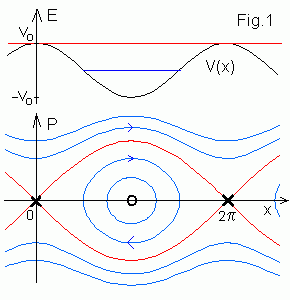# Nonlinear resonance

Here universality of the standard map dynamics is motivated. At first we remind nonlinear pendulum dynamics. Then appearance of resonances in nonlinear systems and single resonance approximation are explained briefly. You can read this in any textbook and go directly to the Standard map.

## Nonlinear pendulum

Hamiltonian and equations of motion for nonlinear pendulum are
H(p,x) = p2/2 + V(x) = p2/2 + Vo cos x = E = const
dx/dt = ∂H/∂p = p ,     dp/dt = -∂H/∂x = Vo sin x
.When -Vo < E < Vo particle oscillates between two turning points. On the phase plane (x, p) its orbit rotates periodically (blue oval curves to the left) around elliptic fixed point O (equilibrium position at x = π ). For E > Vo orbits pass over maxima of V(x) and go to infinity. The motion is unbounded. As since V(x) is periodic, therefore we can consider motion on a cylinder and join the 0 and points. The red separatrix (or homoclinic orbit) at E = Vo separates these two regions. It goes out and come to hyperbolic points X (unstable equilibrium positions at x = 2pn ). Dynamics near homoclinic orbits is very sensitive to perturbations because a small force can throw orbit over (or under) maximum of V(x) and change qualitatively its motion. The "width" of the homoclinic orbit is
max Δp = 4 V o½.

## Resonances

For canonical action-angle variables (I, θ) Hamiltonian H(I) depends on I only and equations of motion are
dI/dt = -∂H(I)/∂θ = 0 ,     I = const ,
dθ/dt = ∂H(I)/∂I = ω(I) = const
.
Under a periodic perturbation V(I, θ, t) = V(I, θ, t + T)
H(I, θ, t) = Ho(I) + εV(I, θ, t) = Ho(I) + ε∑ n,m Vnm(I) einθ - imΩt ,
dI/dt = -∂ H/∂ θ = -iε∑ n,m nVnm(I) einθ - imΩt
,     (*)
dθ/dt = ∂ H/∂ I .
where Ω = 2π /T. For small ε we will search solution as a series
I = I o + εI 1 + ... ,   θ = θ o + εθ 1 + ... ,
I o = const,   θ o = ω(I o)t .

After substitution I o, θ o into (*) we get
dI 1/dt = -i∑ n,m nVnm(I o) exp[i(nω(I o) - mΩ)t] ,
I 1 = -∑ n,m nVnm(I o) /[nω(I o) - mΩ]   ei[nω(I o) - mΩ]t + const
.
At a resonance nω(I) - mΩ = δnm ~ 0 , I 1 and θ 1 contain divergent terms with small denominators Vnmnm. In nonlinear systems frequency ω(I) depends on I, therefore we can get resonanses at any external frequency Ω for some I, n, m.

## Single resonance approximation. Universal Hamiltonian

To avoid this divergence for a single resonance we can solve (*) taking into account only secular terms with nω - mΩ ~ 0
dI/dt = εnVnm sin(nθ - mΩt + φ) ,
dθ/dt = ω(I) + ε dVnm/dI cos(nθ - mΩt + φ)
.
Corresponding Hamiltonian is
H = Ho(I) + εVnm(I) cos(ψ),   ψ = nθ - mΩt + φ.
For small ΔI = I - Io it turns into the nonlinear pendulum Hamiltonian
H(ΔI, ψ) = nω' ΔI 2/2 + nεV cos( ψ ) ,     ω' = dω/dI .
Thus independently on ω(I), V(I) dynamics near resonance is described approximately by this universal Hamiltonian. Resonant terms lead to appearence of homoclinic orbits on the (ψ, ΔI) phase plane (similar to Fig.1) with the width
max ΔI = 4 (εV/|ω'|)1/2 .
Nonresonant terms lead to chaotic dynamics near homoclinic orbits. To see this we can plot Poincare sections of the flow at the moments t = n T . One model example is the standard map below. You see resonances, invariant circles and chaos near homoclinic orbits.

Contents   Previous: Strange attractors   Next: The Standard map
updated 26 August 2003